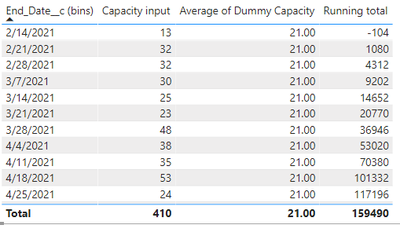cancel
Showing results for
Did you mean:

Fabric is Generally Available. Browse Fabric Presentations. Work towards your Fabric certification with the Cloud Skills Challenge.Regular Visitor

## Trying to calculate a running total column

Hi,

I'm trying to calculate the running total for a table that has two columns Capacity input and Dummy Capacity. The total for the row should be Capacity input - dummy capacity. Example: line 1 in the table below should be 13 - 21 = -8.

Capacity input is the count of line items for each bin and Dummy Capacity is the average capacity per bin (this number can be hardcoded if it's going to make the calculation easier).

I looked around for a few ideas on how to write a DAX that could work, but I haven't been successful so far (you can see the output in the table below).

Here is the DAX:

``````Running total = calculate(
sumx(
Project,
sum(Project[Capacity input])-AVERAGE(Project[Dummy Capacity])
),
filter(
ALLSELECTED(Project),
Project[End_Date__c]<=MAX(Project[End_Date__c])
)
)``````

The table below should give me:

Row 1 = 13 - 21 = -8

Row 2 = -8 +32 - 21 = +19

Row 3 = 19 + 32 -21 = +30Thanks in advance for any help that you can provide.

Best,

Jose

1 ACCEPTED SOLUTIONSuper User

@steamexpert , Try with these changes

Running total = calculate(
sumx(
values(Project[End_Date__c bins]),
calculate(um(Project[Capacity input])-AVERAGE(Project[Dummy Capacity]))
),
filter(
ALLSELECTED(Project),
Project[End_Date__c]<=MAX(Project[End_Date__c])
)
)

2 REPLIES 2Super User

@steamexpert , Try with these changes

Running total = calculate(
sumx(
values(Project[End_Date__c bins]),
calculate(um(Project[Capacity input])-AVERAGE(Project[Dummy Capacity]))
),
filter(
ALLSELECTED(Project),
Project[End_Date__c]<=MAX(Project[End_Date__c])
)
)Regular Visitor

This worked like a charm, thank you @amitchandakAnnouncements#### Power BI Monthly Update - November 2023

Check out the November 2023 Power BI update to learn about new features.#### Fabric Community News unified experience

Read the latest Fabric Community announcements, including updates on Power BI, Synapse, Data Factory and Data Activator.#### Exclusive opportunity for Women!

Join us for a free, hands-on Microsoft workshop led by women trainers for women where you will learn how to build a Dashboard in a Day!#### The largest Power BI and Fabric virtual conference

130+ sessions, 130+ speakers, Product managers, MVPs, and experts. All about Power BI and Fabric. Attend online or watch the recordings.Top Solution Authors
Top Kudoed Authors
Users online (4,235)